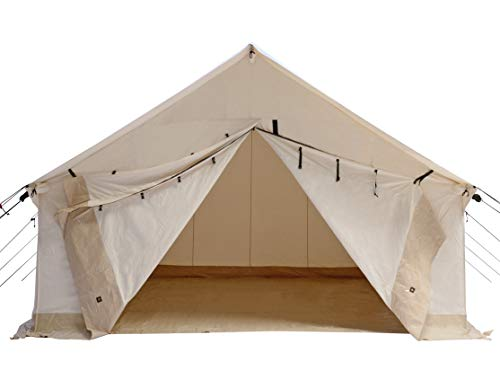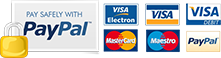# How to calculate the total surface area of a canvas wall tent with four sides 12 x 10 feetTo calculate the total surface area of a wall tent with four sides, we need to determine the area of each side and then sum them up.

Assuming the wall tent has a rectangular shape, with dimensions 12 feet by 10 feet, we can calculate the surface area as follows:

Calculate the area of one of the longer sides: Area of one longer side = length × height = 12 ft × 10 ft = 120 square feet.

Calculate the area of the other longer side (which is identical to the first one): Area of the second longer side = 120 square feet.

Calculate the area of one of the shorter sides: Area of one shorter side = width × height = 10 ft × 10 ft = 100 square feet.

Calculate the area of the other shorter side (which is identical to the first one): Area of the second shorter side = 100 square feet. Sum up the areas of all four sides to get the total surface area: Total surface area = 120 sq ft + 120 sq ft + 100 sq ft + 100 sq ft = 440 square feet.

Therefore, the actual surface area of a 12' x 10' wall tent with four sides is 440 square feet.

Based on a 12' x 10' wall tent, with a total surface area of 440 square feet, you would need approximately two bottles of the Dry Guy Tent and Outdoor Fabric Waterproofing Concentrate (473 ml or 16 oz per bottle) to treat the entire tent. Each bottle of concentrate is estimated to cover 240-300 square feet, so two bottles should provide sufficient coverage for your 12' x 10' wall tent.